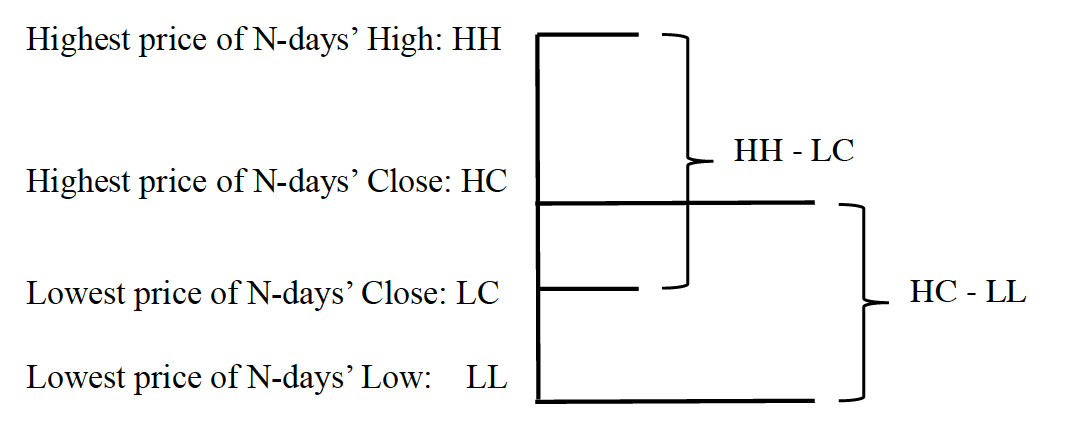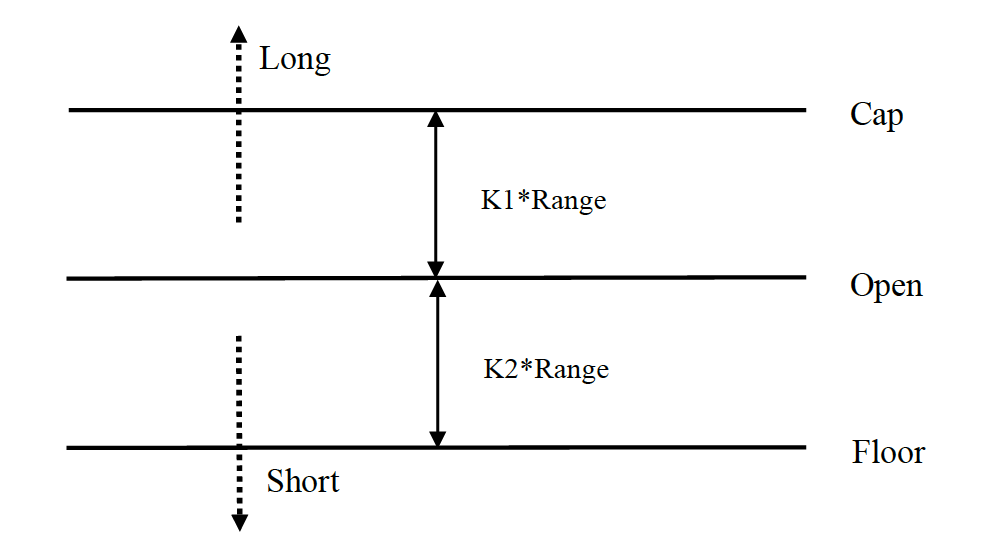## Abstract

The Dual Thrust trading algorithm is a famous strategy developed by Michael Chalek. It has been commonly used in Futures, Forex and Equity markets. The idea of Dual Thrust is similar to a typical breakout system, however dual thrust uses the historical price to construct update the look back period - theoretically making it more stable in any given period.

In this tutorial we give a brief introduction to the strategy and show how to implement this algorithm on QuantConnect. After pulling in the historical price of the chosen stock, the range is calculated based on the close, high, and low over the most recent $$N$$ days. A position is opened when the market moves a certain range from the opening price. We tested the strategy on individual stocks under two market states: a trending market and range bound market.  The results suggest this momentum trading system works better in trending market but will trigger some fake buy and sell signals in much more volatile market. Under the range bound market, we can adjust the parameters to get better return. As a comparison of individual stocks, we also implemented the strategy on SPY. The result suggested that the strategy beat the market.

## Method

#### Step 1 : Initialization of algorithm

Firstly we need to initialize the data and resolution required, as well as the cash and start-end dates for your algorithm. All algorithms must be initialized.

def Initialize(self):
self.SetStartDate(2004, 1, 1)
self.SetEndDate(2017, 8, 30)
self.SetCash(100000)
self.syl = equity.Symbol


Although this is an intraday strategy, we still want to test the strategy within a long period not just within one day. The Scheduled Events methods sets up an function to fire at a specific date or time.

self.Schedule.On(self.DateRules.EveryDay(self.syl),self.TimeRules.AfterMarketOpen(self.syl,0),Action(self.SetSignal))


Here Schedule.On(DateRules, TimeRules, Action()), will trigger every trading day for our stock, at market open. Events are scheduled using date and time rules. Date rules specify on what dates and event will fire. Time rules specify at what time on those dates the event will fire. Inside of the scheduled function, we can pull the recent historical data and current open price each trading day.

#### Step 2:  Implementation of algorithmIn order to calculate the range, each trading day we need the close, high, and low price data over the most recent $$N$$ days. In addition, the open price of the current day is required in order to generate the signals. QuantConnect provides a History(Symbol, TimeSpan, Resolution) method to get the history data like open, close, high, and low for all configured securities over the requested $$N$$-days time span. Then the range is calculated by

$range = max(HH-LC, HC-LL)$

In this implementation, we choose $$N=4$$. It is less than one week and the range would reflect the recent price change.

self.high = []
self.low = []
self.close = []
history = self.History(4, Resolution.Daily)
for slice in history:
bar = slice[self.syl]
self.high.append(bar.High)
self.low.append(bar.Low)
self.close.append(bar.Close)


The long signal is calculated by

$cap = open + K_1 \times range$

The short signal is calculated by

$floor = open – K_2 \times range$

where $$K1$$ and $$K2$$ are the parameters. When $$K1 > K2$$, it is much easier to trigger the long signal and vice versa. For demonstration, here we choose $$K1 = K2 = 0.5$$. In live trading, we can still use historical data to optimize those parameters or adjust the parameters according to the market trend. $$K1$$ should be small than $$K2$$ if you are bullish on the market and $$K1$$ should be much bigger if you are bearish on the market.This system is a reversal system, so if the investor holds a short position when the price breaks the cap line, the short margin should be liquidated first before opening a long position. If the investor holds a long position when the price breaks the floor line, the long margin should be liquidated first before opening a new short position.

holdings = self.Portfolio[self.syl].Quantity
if self.Portfolio[self.syl].Price >= self.selltrig:
if holdings >= 0:
self.SetHoldings(self.syl, 1)
else:
self.Liquidate(self.syl)
self.SetHoldings(self.syl, 1)
elif self.Portfolio[self.syl].Price < self.selltrig: if holdings >= 0:
self.Liquidate(self.syl)
self.SetHoldings(self.syl, -1)
else:
self.SetHoldings(self.syl, -1)


## Conclusion

We tested this strategy on the S&P 500 ETF SPY from 2004 to 2017. During the backtesting period, SPY resulted in a Sharpe Ratio of -0.17 and the drawdown is 41.1%. This is just a basic implementation and the parameters $$K1$$ and $$K2$$ are fixed. The strategy can be further extended to Futures and Forex markets. When $$K1 < K2$$, buy signals are prone to trigger and vice versa. To improve the model we can apply technical indicators to judge the trend of price and adjust the value of two parameters dynamically and apply risk control in the position sizing.

## Reference

1. Gang Wei(May 2012). Dual Thrust Intraday Strategy,  Online Copy (Chinese)